# NEET Chemistry Haloalkanes and Haloarenes Questions SolvedWhen CH3CH2CHCl2 is treated with NaNH2, the product formed is

(a) CH3-CH=CH2                     (b) CH3-C$\equiv$CH

(c) CH3CH2CH${<}_{{\mathrm{NH}}_{2}}^{{\mathrm{NH}}_{2}}$                  (d) CH3CH2C${<}_{{\mathrm{NH}}_{2}}^{\mathrm{Cl}}$

Concept Videos :-

#1 | Preparation of Haloalkane:I
#2 | Preparation of Haloalkane:II
#3 | Properties of Haloalkane:I
#4 | Properties of Haloalkane:II
#7 | Reactions of Dihalides:I
#8 | Preparation of Haloarenes:I
#9 | Properties of Haloarenes:I

Concept Questions :-

Physical & Chemical Properties

(b) CH3-CH2-CHCl2 $\underset{∆}{\overset{{\mathrm{NaNH}}_{2}\left(\mathrm{sodamide}\right)}{\to }}$CH3-CH=CHCl$\underset{∆}{\overset{{\mathrm{NaNH}}_{2}}{\to }}$CH3-C$\equiv$CH

final product

Difficulty Level:

• 19%
• 41%
• 28%
• 14%
Crack NEET with Online Course - Free Trial (Offer Valid Till September 22, 2019)

Chlorobenzene reacts with Mg in dry ether to give a compound (A) which further reacts with ethanol to yield

(a) phenol                           (b) benzene

(c) ethyl benzene                (d) phenyl ether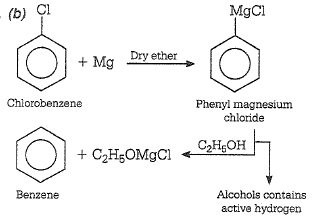Difficulty Level:

• 22%
• 43%
• 26%
• 11%
Crack NEET with Online Course - Free Trial (Offer Valid Till September 22, 2019)

R-CH2-CCl2-R $\stackrel{\mathrm{Reagent}}{\to }$R-C$\equiv$C-R. The reagent is

(a) Na                                   (b) HCl in H2O

(c) KOH in C2H5OH                (d) Zn in alcohol

Concept Videos :-

#1 | Preparation of Haloalkane:I
#2 | Preparation of Haloalkane:II
#3 | Properties of Haloalkane:I
#4 | Properties of Haloalkane:II
#7 | Reactions of Dihalides:I
#8 | Preparation of Haloarenes:I
#9 | Properties of Haloarenes:I

Concept Questions :-

Physical & Chemical Properties

(c) Dehydrohalogenation reaction,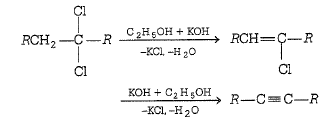In presence of ethanolic KOH substrate gives an elimination reaction.

Difficulty Level:

• 18%
• 11%
• 59%
• 14%
Crack NEET with Online Course - Free Trial (Offer Valid Till September 22, 2019)

Ethyl chloride is converted into diethyl ether by

(a) Wurtz reaction                           (b) Grignard reaction

(c) Perkin's reaction                         (d) Williamson's synthesis

(d) C2H5Cl - NaOC2H5 $\to$C2H5-O-C2H5 + NaCl

It is an example of Williamson's synthesis. Limitations of Williamson's synthesis if 3$°$ alkyl halide used in reaction then alkene formed.

Difficulty Level:

• 28%
• 10%
• 14%
• 49%
Crack NEET with Online Course - Free Trial (Offer Valid Till September 22, 2019)

The alkyl halide is converted into an alcohol by

(a) addition                       (b) substitution

(c) dehydrohalogenation     (d) elimination

Concept Videos :-

#1 | Preparation of Haloalkane:I
#2 | Preparation of Haloalkane:II
#3 | Properties of Haloalkane:I
#4 | Properties of Haloalkane:II
#7 | Reactions of Dihalides:I
#8 | Preparation of Haloarenes:I
#9 | Properties of Haloarenes:I

Concept Questions :-

Physical & Chemical Properties

(b) RCl + NaOH(aq) $\to$ROH + NaCl

It is an example of nucleophilic substitution reaction.

Difficulty Level:

• 8%
• 69%
• 18%
• 7%
Crack NEET with Online Course - Free Trial (Offer Valid Till September 22, 2019)

Addition of Br2 on cis-butene-2 gives:

(a) a racemic mixture of 2,3-dibromobutane

(b) meso form of 2,3-dibromobutane

(c) dextro form of 2,3-dibromobutane

(d) laevo form of 2,3-dibromobutane

Concept Videos :-

Concept Questions :-

Isomer and Chirality

Difficulty Level:

• 64%
• 26%
• 9%
• 3%
Crack NEET with Online Course - Free Trial (Offer Valid Till September 22, 2019)

The number of different substitution products possible when ethane is allowed to react with bromine in sunlight are:

(a) 9           (b) 6

(c) 8           (d) 5

Concept Videos :-

Concept Questions :-

Isomer and Chirality

There are nine isomers:

Difficulty Level:

• 33%
• 38%
• 15%
• 17%
Crack NEET with Online Course - Free Trial (Offer Valid Till September 22, 2019)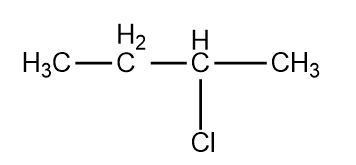, Cl obtained by chlorination of n-butane, will be

(a) meso form              (b) racemic mixture

(c) d-form                 (d) l-form

Concept Videos :-

Concept Questions :-

Mechanism of Substitution Reactions

(b) Chlorination of n-butane takes place by free radical mechanism as follows: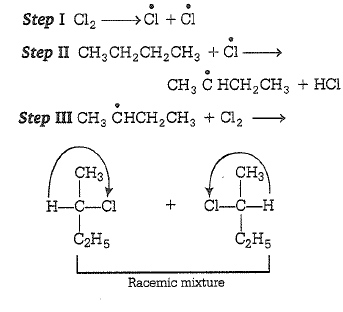Difficulty Level:

• 17%
• 70%
• 10%
• 5%
Crack NEET with Online Course - Free Trial (Offer Valid Till September 22, 2019)

In ${\mathrm{S}}_{{\mathrm{N}}^{2}}$ reactions, the correct order of reactivity for the following compounds : ${\mathrm{CH}}_{3}\mathrm{Cl}$${\mathrm{CH}}_{3}{\mathrm{CH}}_{2}\mathrm{Cl}$${\left({\mathrm{CH}}_{3}\right)}_{2}\mathrm{CHCl}$ and ${\left({\mathrm{CH}}_{3}\right)}_{3}\mathrm{CCl}$ is :

(a) ${\mathrm{CH}}_{3}\mathrm{Cl}$>${\left({\mathrm{CH}}_{3}\right)}_{2}\mathrm{CHCl}$>${\mathrm{CH}}_{3}{\mathrm{CH}}_{2}\mathrm{Cl}$>${\left({\mathrm{CH}}_{3}\right)}_{3}\mathrm{CCl}$

(b) ${\mathrm{CH}}_{3}\mathrm{Cl}$>${\mathrm{CH}}_{3}{\mathrm{CH}}_{2}\mathrm{Cl}$>${\left({\mathrm{CH}}_{3}\right)}_{2}\mathrm{CHCl}$>${\left({\mathrm{CH}}_{3}\right)}_{3}\mathrm{CCl}$

(c) ${\mathrm{CH}}_{3}{\mathrm{CH}}_{2}\mathrm{Cl}$>${\mathrm{CH}}_{3}\mathrm{Cl}$>${\left({\mathrm{CH}}_{3}\right)}_{2}\mathrm{CHCl}$>${\left({\mathrm{CH}}_{3}\right)}_{3}\mathrm{CCl}$

(d) ${\left({\mathrm{CH}}_{3}\right)}_{2}\mathrm{CHCl}$>${\mathrm{CH}}_{3}{\mathrm{CH}}_{2}\mathrm{Cl}$>${\mathrm{CH}}_{3}\mathrm{Cl}$>${\left({\mathrm{CH}}_{3}\right)}_{3}\mathrm{CCl}$

Concept Videos :-

Concept Questions :-

Mechanism of Substitution Reactions

(b) Due to more -I.E. of attached groups in ${\left({\mathrm{CH}}_{3}\right)}_{3}\mathrm{CCl}$ or due to max crowding round central carbon atom favours ${\mathrm{S}}_{\mathrm{N}}$ l mechanism.

Difficulty Level:

• 11%
• 76%
• 10%
• 5%
Crack NEET with Online Course - Free Trial (Offer Valid Till September 22, 2019)

Action of RMgX with vinyl chloride gives:

(a) alkane                                  (b) alkyne

(c) alkene                                  (d) all of these

(c) RMgX +$\mathrm{ClHC}={\mathrm{CH}}_{2}\to \mathrm{RCH}={\mathrm{CH}}_{2}$ +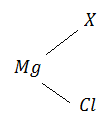Difficulty Level:

• 28%
• 12%
• 44%
• 17%
Crack NEET with Online Course - Free Trial (Offer Valid Till September 22, 2019)